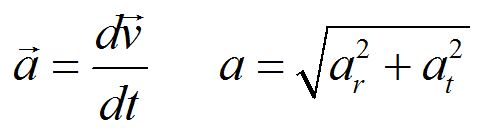PH 212

# Motion in a plane

• 2-D dynamics
• Uniform circular motion
• Circular orbits
• Fictitious forces
• Nonuniform circular motion

Newton's second law is a vector equation, so it can be broken into components.In this section, we will address motion in a plane, with particular focus on circular motion. In general, an object can be spiraling in a third dimension. In 2-D motion, the motion of the object is constrained to a plane, so we need only consider acceleration tangential to the circle and radial acceleration. Even in the case of uniform circular motion, where the speed of the object is constant, radial acceleration is not zero because the velocity vectors are changing direction.

The diagrams below show velocity vectors of objects in uniform circular motion. Please rank their radial accelerations.For uniform circular motion, the speed is constant, even though the velocity vectors change. For non-uniform circular motion, the speed is not constant.In both cases, the equation for radial acceleration is true at any point in time.To find the magnitude of the total acceleration, the radial and tangential components must be added as vectors.